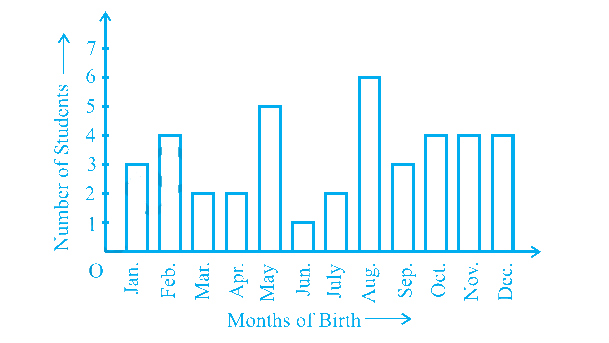# Ex.15.1 Q3 Probability Solution - NCERT Maths Class 9

Go back to  'Ex.15.1'

## Question

Refer to Example 5, Section 14.4, Chapter 14. Find the probability that a student of the class was born in August.

In a particular section of Class IX, $$40$$ students were asked about the months of their birth and the following graph was prepared for the data so obtained. Find the probability that a student of the class was born in August.Video Solution
Probability
Ex exercise-15-1 | Question 3

## Text Solution

What is known?

Total number of students in class and number of students born in each month.

What is unknown?

Probability of students born in the month of August.

Reasoning:

The empirical probability $$P(E)$$ of an event $$E$$ happening, is given by:

\begin{align}{P}({E})=\frac{ \begin{bmatrix} \text { Number of trials in which }\\ \text{the event happened } \end{bmatrix} }{ \begin{bmatrix} \text { The total number} \\ \text{of trials } \end{bmatrix} }\end{align}

Use probability to derive the solution where

Probability [students born in particular month]

\begin{align} & = \frac{\begin{bmatrix}{\text{No of students born }} \\ {\text{in particular month} }\end{bmatrix}}{\begin{bmatrix} \text{Total number of} \\\text{ students in class} \end{bmatrix}} \end{align}

Steps:

Given total number of students in class $$= 40$$

No of students born in August $$= 6$$

Probability students born in August

\begin{align} & { = \frac{\begin{bmatrix} {\text{No of students born in }} \\ {\text{ the }} {\text{month of August}}\end{bmatrix} } { \begin{bmatrix} \text{Total number of}\\ \text{ students in class } \end{bmatrix}} } \\ &= \frac{{\rm{6}}}{{40}} \\&= \frac{3}{{20}}\end{align}

Learn from the best math teachers and top your exams

• Live one on one classroom and doubt clearing
• Practice worksheets in and after class for conceptual clarity
• Personalized curriculum to keep up with school